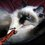# The max and min functions: what are they? A response to Milly Choochoo.

2 months and 3 weeks ago the user Milly Choochoo asked in this thread a question about how to deal with expressions involving max and min functions. Unfortunately, he didn't receive much attention. But I wrote to him an answer, that I will share with you if it can help anyone.

Dear Milly;

What is $\operatorname{max}\{a,b\}$? It's just a function that gives us the biggest number from $a,b$. The same thing for $\operatorname{min}\{a,b\}$, except that this time, it gives us the smallest number from $a,b$. Let's begin with some few examples to wrap our heads around those two functions. From the definitions we got $\operatorname{max}\{-3,8\}$ is equal to $8$. Since out of $-3$ and $8$ it's the latter that is the biggest. And for $\operatorname{min}\{-7,1\}$ we can see that it is equal to $-7$ since this last one is the smallest number out of $-7$ and $1$.

Now that we have understood those functions, we need to determine a way that will reveal to us a formula to find the min and max of any two numbers. Let's take two arbitrary numbers as an example like $x,y$ such that $\displaystyle\underline{x\lt y}$, which is a very important assumption for what will come. If we graph those into the real number line then we will see that $x$ is in the left in regards of $y$ as shown in this image:http://i.stack.imgur.com/OpjU2.png

One of the numbers we can define based on $x$ and $y$ is the their average, $(x+y)/2$. What this average graphically means, is that it represent the number whose corresponding point on the number line is a midpoint of the segment $[x,y]$, as this image show:http://i.stack.imgur.com/x10g3.png

We've made some neat progress till now, but we'll get to the next point only by seeing that this midpoint unlocks to us a new possibility. This midpoint makes an axis of symmetry, and so with the rightly chosen way we can go from the quantity $(x+y)/2$ to the smallest number, that is $x$, or to the biggest number $y$. To see what I mean look carefully at this image:http://i.stack.imgur.com/ozkyR.png

So by adding the distance from $(x+y)/2$ to $y$ to $(x+y)/2$ itself we will obtain $y$. And if we substract the distance from $(x+y)/2$ to $x$ to $(x+y)/2$ itself we will obtain $x$. So we actually found a way to get $x$ and $y$ out of some formula that will soon discover. Note however that those two distances are the same. And how is distance represented? I mean how to find the distance from $a$ to $b$? We use absolute values! To get: $\text{distance from }a\text{ to }b=|a-b|.$ So if we use that property we will get that:

\eqalign{ \operatorname{max}\{x,y\}&=\dfrac{x+y}{2}+\left|\dfrac{x+y}{2}-y\right|\\ &=\dfrac{x+y}{2}+\left|\dfrac{x+y-2y}{2}\right| \\ &=\dfrac{x+y}{2}+\left|\dfrac{x-y}{2}\right| \\ &=\dfrac{x+y}{2}+\left|\dfrac{1}2(x-y)\right| \\ &=\dfrac{x+y}{2}+\dfrac12\left|(x-y)\right| \quad\text{using properties of the }|\,\cdot\,| \\ &=\dfrac{x+y}{2}+\dfrac{\left|(x-y)\right|}2 \\ &=\dfrac{x+y+\left|x-y\right|}2\quad\blacksquare \\ }

Now I'm sure you can do the same yourself to prove that:

$\operatorname{min}\{x,y\}=\dfrac{x+y-\left|x-y\right|}2.$

So now whenever you see a problem involving $\operatorname{min}\{x,y\}$ or $\operatorname{max}\{x,y\}$ just replace it with the formulas we found above, and the rest would be easy.

## Exercises:

1) Prove that $\operatorname{min}\{x,x\}=\operatorname{max}\{x,x\}=x.$

2) Derive a formula for $\operatorname{min}\{x,y,z\}$ and $\operatorname{max}\{x,y,z\}.$

I hope this helps. Best wishes, $\cal H$akim.Note by حكيم الفيلسوف الضائع
6 years, 3 months ago

This discussion board is a place to discuss our Daily Challenges and the math and science related to those challenges. Explanations are more than just a solution — they should explain the steps and thinking strategies that you used to obtain the solution. Comments should further the discussion of math and science.

When posting on Brilliant:

• Use the emojis to react to an explanation, whether you're congratulating a job well done , or just really confused .
• Ask specific questions about the challenge or the steps in somebody's explanation. Well-posed questions can add a lot to the discussion, but posting "I don't understand!" doesn't help anyone.
• Try to contribute something new to the discussion, whether it is an extension, generalization or other idea related to the challenge.

MarkdownAppears as
*italics* or _italics_ italics
**bold** or __bold__ bold
- bulleted- list
• bulleted
• list
1. numbered2. list
1. numbered
2. list
Note: you must add a full line of space before and after lists for them to show up correctly
paragraph 1paragraph 2

paragraph 1

paragraph 2

[example link](https://brilliant.org)example link
> This is a quote
This is a quote
    # I indented these lines
# 4 spaces, and now they show
# up as a code block.

print "hello world"
# I indented these lines
# 4 spaces, and now they show
# up as a code block.

print "hello world"
MathAppears as
Remember to wrap math in $$ ... $$ or $ ... $ to ensure proper formatting.
2 \times 3 $2 \times 3$
2^{34} $2^{34}$
a_{i-1} $a_{i-1}$
\frac{2}{3} $\frac{2}{3}$
\sqrt{2} $\sqrt{2}$
\sum_{i=1}^3 $\sum_{i=1}^3$
\sin \theta $\sin \theta$
\boxed{123} $\boxed{123}$

Sort by:

Out of topic, are you Hakim on MSE?

- 6 years, 1 month ago

Yeah, and you are Tunk-Fey on MSE.

- 5 years, 11 months ago

Thank you!

- 6 years, 2 months ago

Well done Champ

- 6 years, 3 months ago

Great note!!! Thanks.... :)

- 6 years, 3 months ago

Nice approach .. :)

- 6 years, 3 months ago